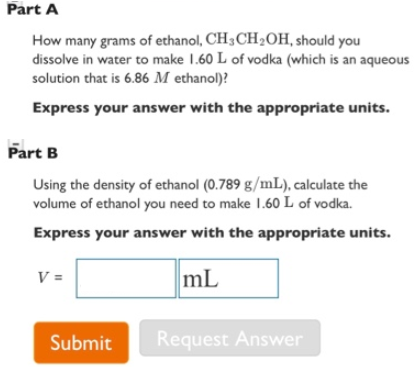# Problem: Part A How many grams of ethanol, CH3CH2OH, should you dissolve in water to make 1.60 L of vodka (which is an aqueous solution that is 6.86 M ethanol)? Expess your answer with the appropriate units.Part B Using the density of ethanol (0.789 g/mL), calculate the volume of ethanol you need to make 1.60 L of vodka. Express your answer with the appropriate units.

###### FREE Expert Solution
87% (106 ratings)###### Problem Details

Part A

How many grams of ethanol, CH3CH2OH, should you dissolve in water to make 1.60 L of vodka (which is an aqueous solution that is 6.86 M ethanol)?

Part B

Using the density of ethanol (0.789 g/mL), calculate the volume of ethanol you need to make 1.60 L of vodka.